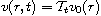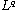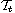Electron. J. Differential Equations, Vol. 2018 (2018), No. 139, pp. 1-13.

### Maximal estimates for fractional Schrodinger equations with spatial variable coefficient Bo-Wen Zheng

Abstract:
Letbe the solution to a fractional Schrodinger equation where the coefficient of Laplacian depends on the spatial variable. We prove some weightedestimates for the maximal operator generated bywith initial data in a Sobolev-type space.

Submitted May 28, 2017. Published July 3, 2018.
Math Subject Classifications: 35B65, 35Q40, 35Q55.
Key Words: Schrodinger equation with spatial variable coefficient; maximal estimate; Hankel-Sobolev space.

Show me the PDF file (289 KB), TEX file for this article.Bo-wen Zheng College of Sciences China Jiliang University Hangzhou 310018, China email: bwen_zj1516@126.com, 17a0802126@cjlu.edu.cn